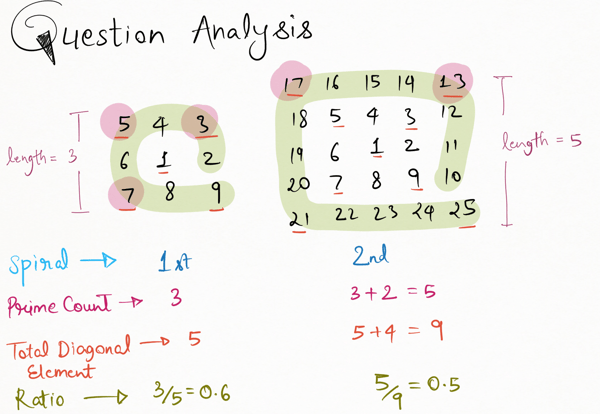Related Tags

communitycreator

# How to solve Project Euler 58

Armaan Nougai

### Problem Statement

The numberS below spiral anti-clockwise starting from 1 in the following manner:

37 36 35 34 33 32 31
38 17 16 15 14 13 30
39 18  5  4  3 12 29
40 19  6  1  2 11 28
41 20  7  8  9 10 27
42 21 22 23 24 25 26
43 44 45 46 47 48 49


If this spiraling continues, what is the length of the side of the square pattern for which the ratio of primes, along both diagonals, falls below 10% for the first time?### Solution Approach

We need the length of the square spiral when the ratio of primes on the diagonals is <10% for the first time.

#### Observations

1. When we perform nth spiral, the total length of the square spiral will be length = 2*n+1.

2. All the values on the down-right diagonal are non-prime; they are squares of odd numbers.

3. The corner elements of the square after nth spiral are v = (2*n-1)**2.

v+(length-1)
v+2*(length-1)
v+3*(length-1)
v+4*(length-1)


### Implementation

• We make a for loop for n spirals.

• For each spiral n, we check how many corner elements are prime and add the number of corner primes to the total prime count.

• Now for any spiral n, the total prime count is such that, prime count <0.1*(length*4+1) , we break the loop and return the total length of the square spiral after this nth spiral i.e., n*2+1.

We also need a fast prime checker, so we go for the Miller-Rabin primality test, which is indeed a probable prime checker only, but this works fine for this question.

### Code

Variables used:

• ratio: Ratio of primes on the diagonals of the square pattern.

• TotalPrimeCnt: Total number of primes on the diagonals of square pattern.

• PrevDownRightCornerValue: Value of the down right corner element for a particular n-1th spiral.

• nthSpiralCornerValues: Corner values of nth spriral.

import random

def func_power(v, c, p):
res = 1

v = v % p
while (c > 0):
if (c & 1):
res = (res * v) % p

c = c>>1
v = (v * v) % p

return res

def miller_test(d, n):
a = 2 + random.randint(1, n - 4)
v = func_power(a, d, n)

if (v == 1 or v == n - 1):
return True

while (d != n - 1):
v = (v * v) % n
d *= 2;

if (v == 1):
return False
if (v == n - 1):
return True
return False

def isPrime(n, k=4):
if (n <= 1 or n == 4):
return False
if (n <= 3):
return True

d = n - 1
while (d % 2 == 0):
d //= 2

for i in range(k):
if (miller_test(d, n) == False):
return False

return True

ratio=100

TotalPrimesCnt = 0
for n in range(1,100000):
PrevDownRightCornerValue = (2*(n-1) + 1)**2

nthSpiralCornerValues = [ PrevDownRightCornerValue+j*(2*n) for j in range(1,4)]
TotalPrimesCnt += sum([isPrime(value) for value in nthSpiralCornerValues])
ratio = TotalPrimesCnt/(n*4+1)

if ratio<0.1:
break

totalLength = n*2 + 1
print(totalLength)



RELATED TAGS

communitycreator

CONTRIBUTOR

Armaan Nougai
RELATED COURSES

View all Courses

Keep Exploring

Learn in-demand tech skills in half the time